# IB DP Physics: SL复习笔记2.3.2 Gravitational Potential Energy

### Gravitational Potential Energy

• Gravitational potential energy (Ep) is energy stored in a mass due to its position in a gravitational field
• If a mass is lifted up, it will gain Ep (converted from other forms of energy)
• If a mass falls, it will lose Ep (and be converted to other forms of energy)
• The equation for gravitational potential energy for energy changes in a uniform gravitational field is: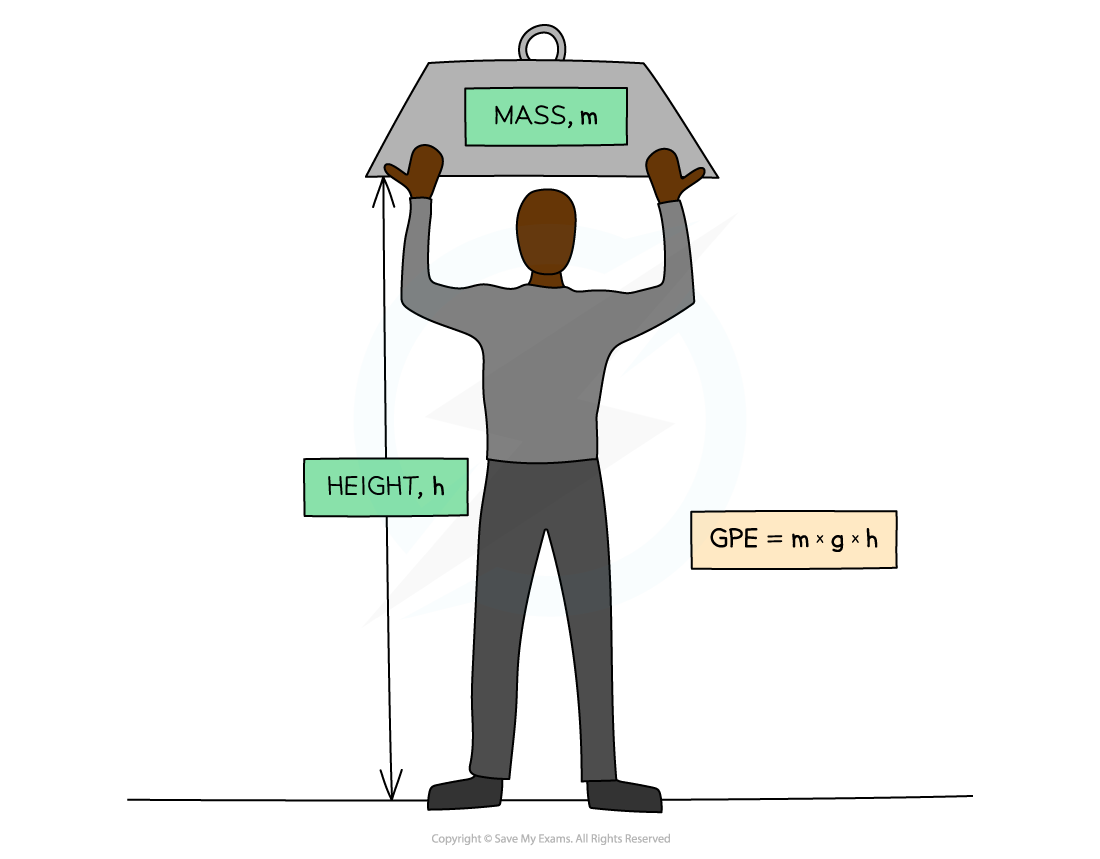Gravitational potential energy (GPE): The energy an object has when lifted up

• The potential energy on the Earth’s surface at ground level is taken to be equal to 0
• This equation is only relevant for energy changes in a uniform gravitational field (such as near the Earth’s surface)

#### Derivation of GPE Equation

• When a heavy object is lifted, work is done since the object is provided with an upward force against the downward force of gravity
• Therefore energy is transferred to the object
• This equation can therefore be derived from the work done#### Worked Example

To get to his apartment a man has to climb five flights of stairs.

The height of each flight is 3.7 m and the man has a mass of 74 kg.

What is the approximate gain in the man's gravitational potential energy during the climb?

A.     13 000 J               B.     2700 J               C.     1500 J               D.     12 500 J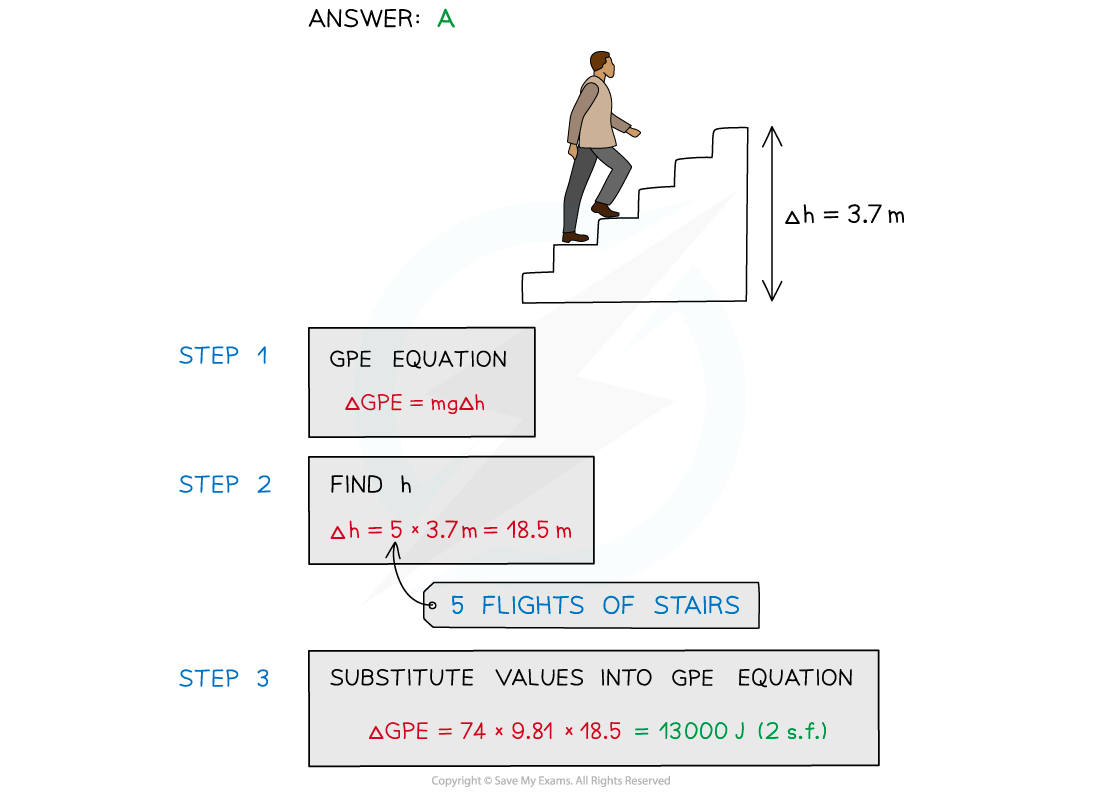#### GPE vs Height

• The two graphs below show how GPE changes with height for a ball being thrown up in the air and when falling down (ignoring air resistance)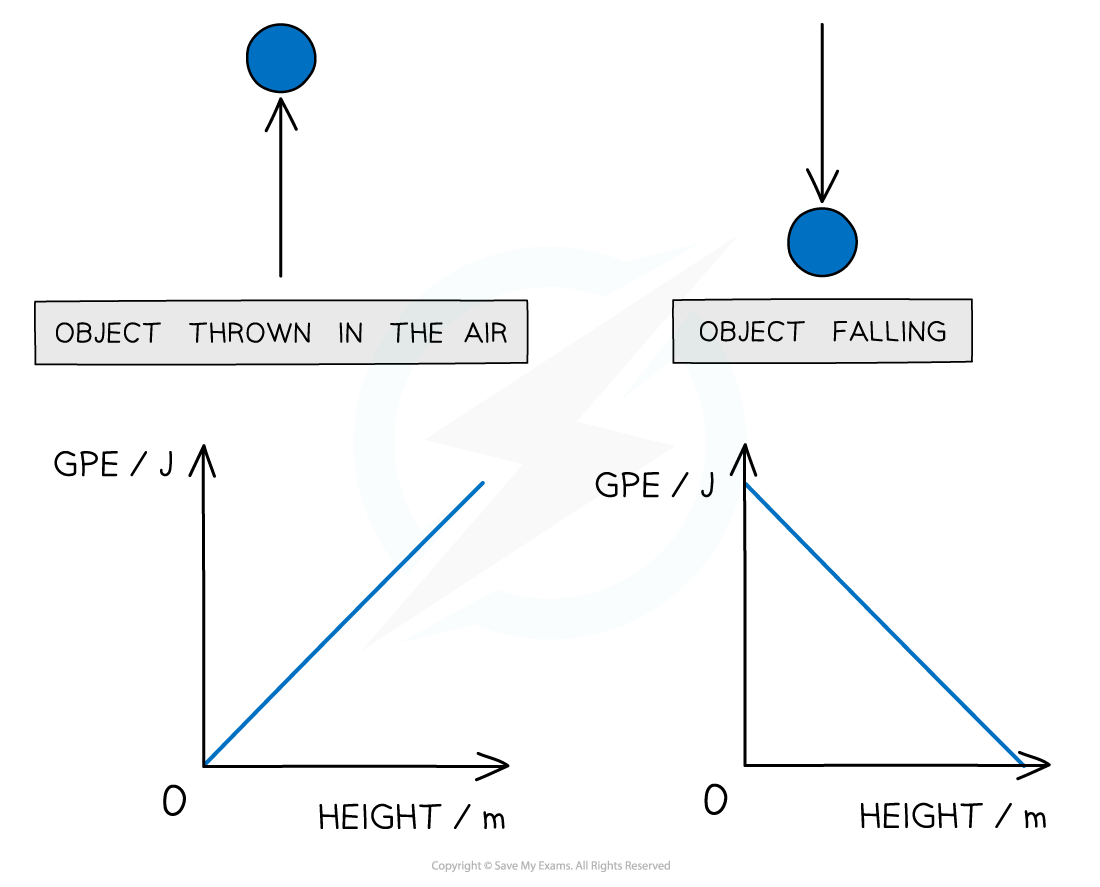Graphs showing the linear relationship between GPE and height

• Since the graphs are straight lines, GPE and height are said to have a linear relationship
• These graphs would be identical for GPE against time instead of height

#### Relationship between GPE & KE

• There are many scenarios that involve the transfer of kinetic energy into gravitational potential, or vice versa
• Some examples are:
• A swinging pendulum
• Objects in freefall
• Sports that involve falling, such as skiing and skydiving
• Using the principle of conservation of energy, and taking any drag forces as negligible:

Loss in potential energy = Gain in kinetic energy

#### Worked Example

The diagram below shows a skier on a slope descending 750 m at an angle of 25° to the horizontal.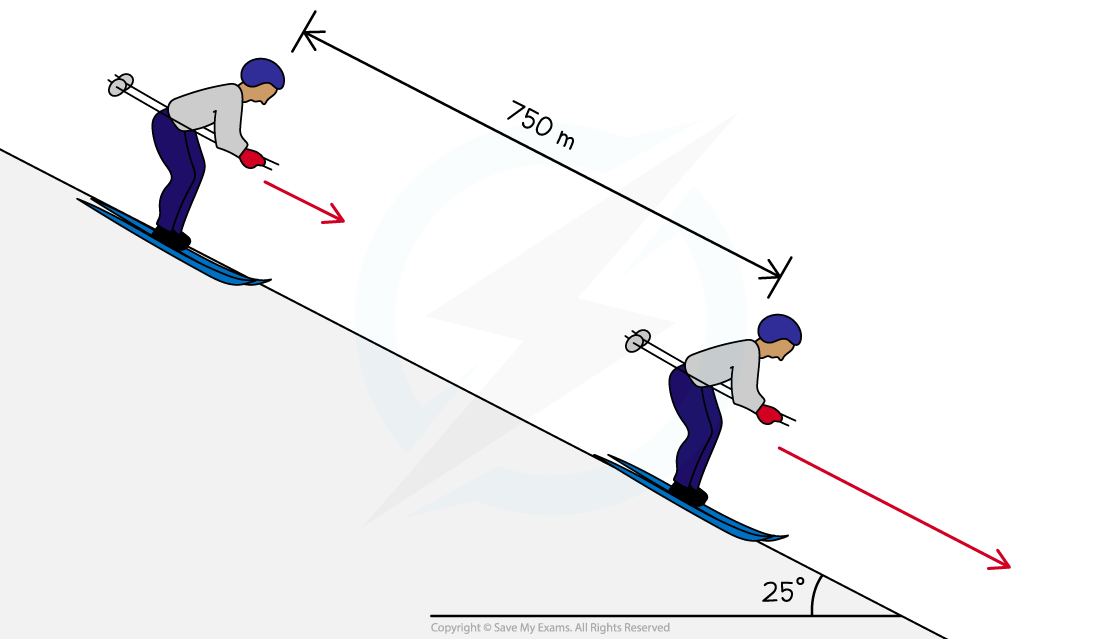Calculate the final speed of the skier, assuming that he starts from rest and 15% of his initial gravitational potential energy is not transferred to kinetic energy.

Step 1: Write down the known quantities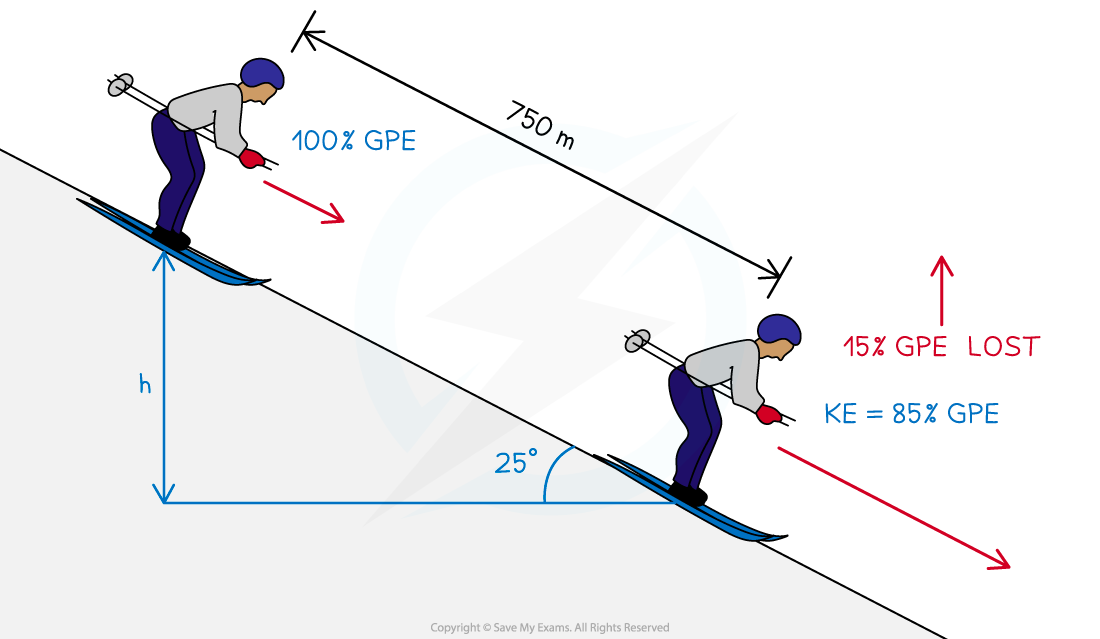• Vertical height, h = 750 sin 25°
• Ek = 0.85 Ep

Step 2: Equate the equations for Ek and Ep

Ek = 0.85 Ep

½ mv2 = 0.85 × mgh

Step 3: Rearrange for final speed, vStep 4: Calculate the final speed, v